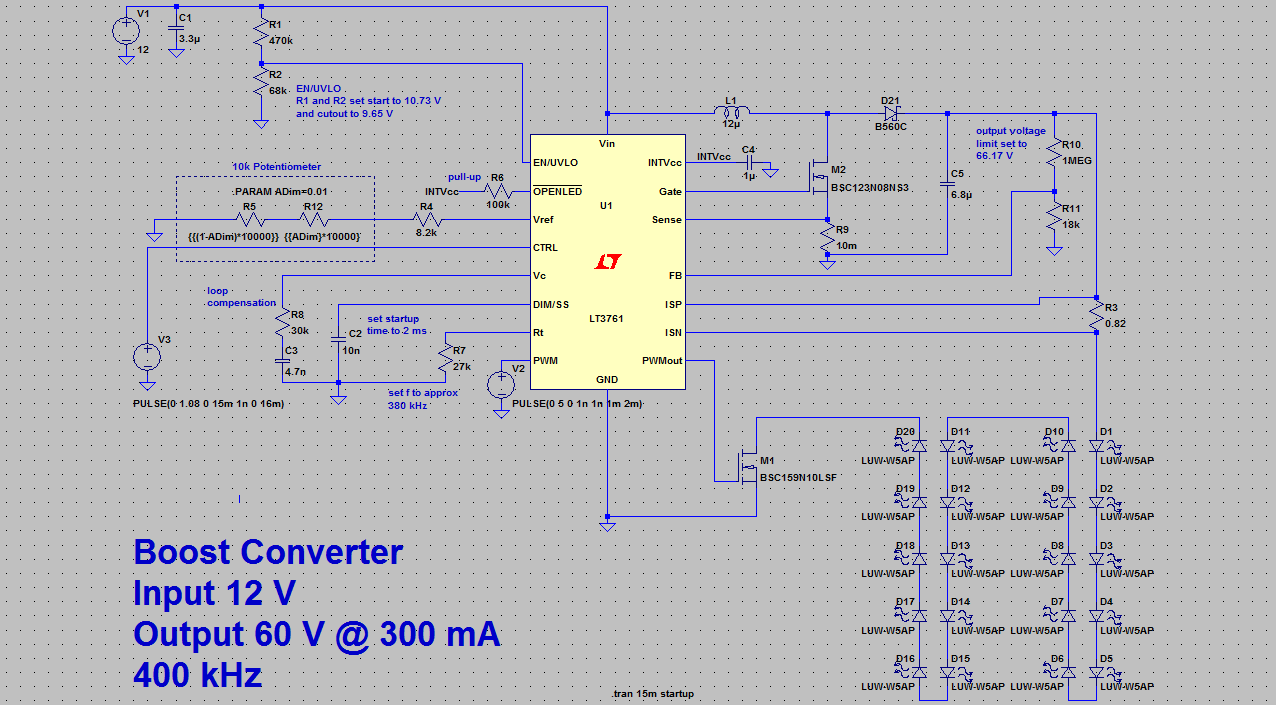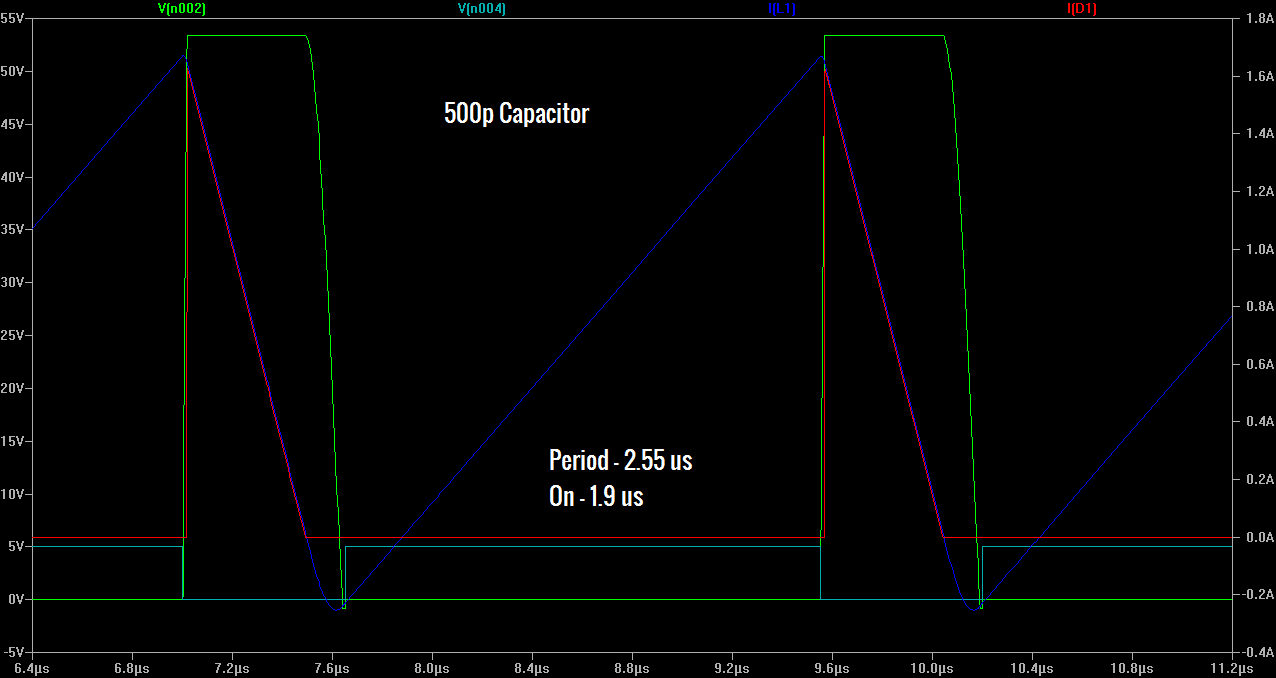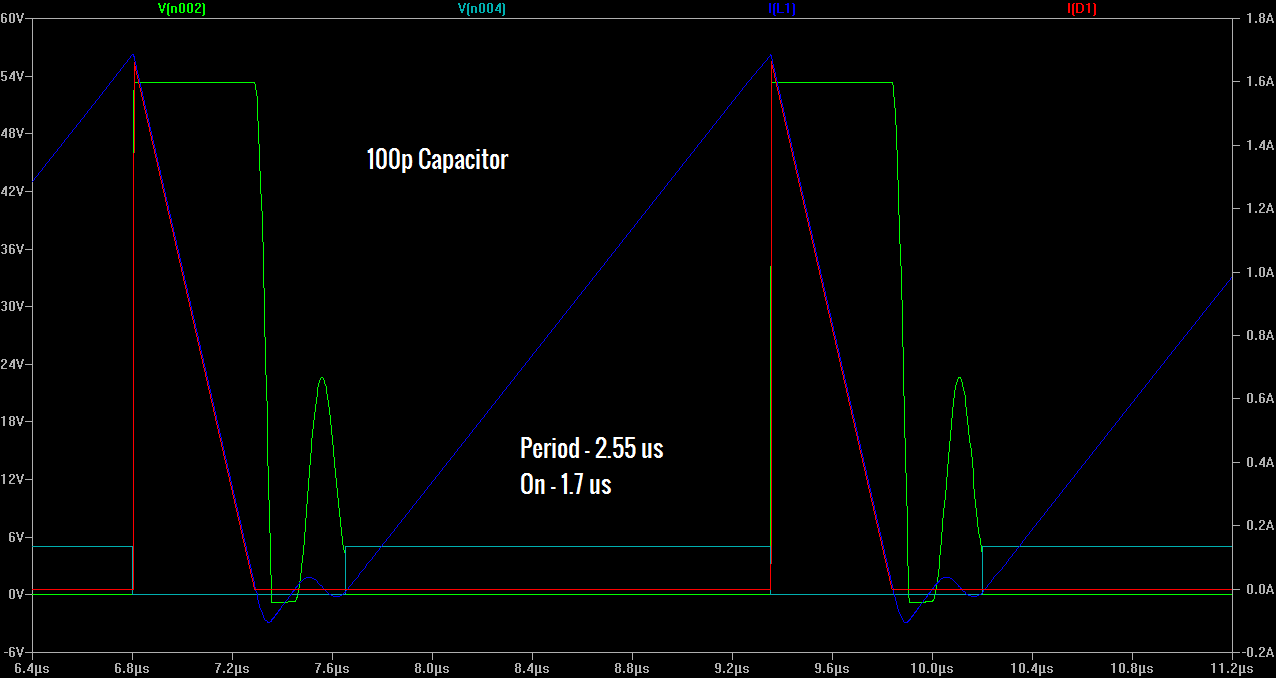## Tuesday, February 10, 2015

### I hope you find this blog enjoyable and helpful. Support it with a small donation.

I've been keeping myself occupied by (slowly) designing a boost converter for an LED light panel, and during one of my simulations I noticed something weird, there was current flowing backwards through the inductor.  I couldn't seem to find any reference to this anywhere so I thought I'd write up what I think is happening.

First I'll give a quick explanation of how a boost converter is meant to work using the schematic below.  When the power mosfet M2 conducts, the input voltage is placed across the inductor L causing the current through it to rise.  When M2 is switched off, the current through the inductor now flows through D21 to fill the capacitor C5 and supply the load.  I won't go into the maths, but it's trivial to calculate the voltage gain of the converter.  It's important to note that current can't flow backwards through the inductor at any point.  For this to happen, current has to either go backwards though the diode D21 (which happens a tiny bit, but isn't what we're seeing) or it has to come out of the mosfet M2, which doesn't make much sense either.Boost converter schematic
The simulation that initially caused me to look into this is below.  It occurs during startup, when the output capacitor isn't fully charged and when the controller is in soft start mode.  At this point the circuit is operating in discontinuous mode, meaning that the inductor current will fall to zero during each switching cycle.  Nearly every textbook will illustrate this with a triangular waveform with a flat section in between triangles to indicate the zero current section.  This isn't what happens though.  I'll step through the stages of operation in the image below.

1. The mosfet is turned on (gate voltage is the green waveform) and the current in the inductor starts to ramp up (light blue).  The voltage at the point between the inductor and mosfet drops to zero (red) and there isn't any current flowing through the diode (dark blue).
2. The mosfet is turned off.  The current flowing through the inductor starts to ramp down.  The voltage at the point between the inductor and mosfet increases to near the output voltage. It can be seen that the inductor current goes through the diode as the dark and light blue plots are the same in this section.
3. This is the point where traditional explanations fail.  The mosfet is still off and the diode current has reached zero.  The voltage between the mosfet and inductor starts to fall as you would expect.
4. The inductor current keeps ramping down though, and in this case reaches around 200 mA in the reverse direction.  The only place this can come from is the mosfet.Boost Converter Simulation
So what's going on here?  I contend that this can be explained by taking the parasitic capacitance of the mosfet and diode into account.  I'll leave exactly what capacitance causes this up to someone more familiar with semiconductor physics.  To demonstrate this and remove other variables I've put together a simplified model below.

The input voltage source remains. As the output capacitor is made increasingly large it starts to act like a voltage source, therefore it can be replaced with a voltage source set to the output voltage we are interested in, and a steady state analysis can be performed.  The mosfet has been replaced by a voltage controlled switch with a parallel capacitance, in this case 500 pF, to represent the parasitic capacitance.  A reverse biased diode has also been placed in parallel to represent the mosfet body diode.  The Schottky diode connected to the output has been replaced with an ideal diode with an appropriate forward voltage.Simplified Model
Note: I apologise for the graphs, you may need to click on them to see things more clearly.  The voltage and current scales don't have a common zero either.

Running this simulation gives an almost identical plot to the one above, but we can now see what is happening more clearly.  The inductor ramps up as before (dark blue), it then ramps down to zero amps and the output diode stops conducting (red), but we can now see that the parasitic capacitance is still charged (green), it starts to discharge, but can only do this via the input inductor.  This is the reverse current through the inductor.  What's happening is that the parasitic capacitance is resonating with the main inductor around the zero volt point.  In the simulation below the resonance occurs at a frequency that makes it look like it's part of normal operation.  The period of resonance for an LC circuit is 2*pi*sqrt(LC), in this case that's equal to approximately 0.5 us.

It's hard to see but you may also notice a tiny delay between the time the mosfet is turned off and when the diode starts to conduct.  This is the first bit of current from the inductor charging the parasitic capacitance.  Once this capacitance is charged and the voltage reaches a high enough point, the diode will then start to conduct.Simplified simulation
All of this can be made clearer by shortening the on period as seen below.  Ideally the time spent with a negative inductor current should be around half a period of the resonance 0.25 us, which is close, but there is another effect at play that can be see in the green trace.  The voltage between the inductor and mosfet hits a limit of -0.7 volts.  At this point the body diode starts to conduct, effectively dampening the resonance.Simplified simulation - reduced on time
If we were to reduce the parasitic capacitance by a factor of 5, the resonance period would be reduced by a factor of sqrt(5), giving 0.22 us.  Which is once again close to the simulation below.  This time the resonance is clearly seen in the voltage between the inductor and mosfet.  It can also be seen where the bottom of a typical resonance waveform has been clamped by the body diode.Simplified simulation - lower capacitance
This is another one of those theoretical cases.  I don't know if this will really happen when a real device is built.  I don't even really know if it's a bad thing, I just had to put an explanation up in case someone else has the same issue.

You can get the related files here.